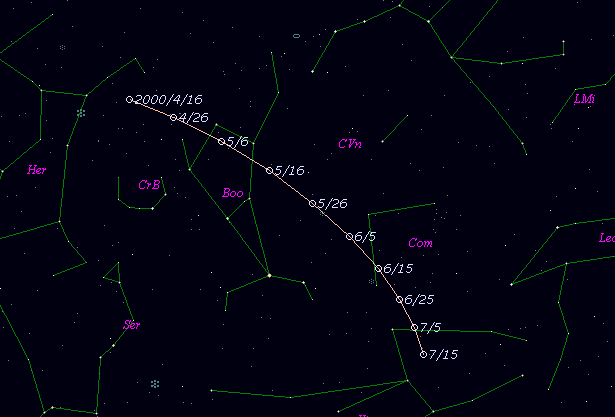# \$B%U%'%j%9WB@1(B

C/2000 J1 ( Ferris )###\$B%W%m%U%#!<%k(B

 \$BH/8+F|(B 2000\$BG/(B5\$B7n(B4\$BF|(B \$BH/8+8wEY(B 19.0\$BEy(B \$BH/8+ W. D. Ferris (LONEOS)

###\$B50F;MWAG(B

```   Improved parabolic orbital elements by K. Muraoka,
from 20 observations  2000 May 4 to 9, m.e. = +/- 0".44.

T  =  2000 May  12.776  TT
Peri. =  147.630
Node  =   28.225   (2000.0)
Incl. =   99.031
q  =    2.55276  AU
e  =    1.0
```

###\$B@1?^(B###\$B8wEYJQ2=(B

```        m1 = 14.0 + 5 log\$B&\$(B + 10.0 log r
```##### \$B50F;MWAG\$OB<2,7r<#;a\$N7W;;\$K\$h\$k\$b\$N\$G\$9!#(B \$B@1?^\$O(B StellaNavigator Ver.2.0 for Windows (\$B%"%9%H%m%"!<%D(B \$BJTCx(B / \$B%"%9%-!<=PHG6I4)(B) \$B\$G:n@.\$7\$?\$b\$N\$G\$9!#(B \$B8wEY%0%i%U\$O(BComet for Windows\$B\$G:n@.\$7\$?\$b\$N\$G\$9!#(B